The word quadrature has (at least) three incompatible meanings. Integration by quadrature either means solving an Integral analytically (i.e., symbolically in terms of known functions), or solving of an integral numerically (e.g., Gaussian Quadrature, Quadrature Formulas). The word quadrature is also used to mean Squaring: the construction of a square using only Compass and Straightedge which has the same Area as a given geometric figure. If quadrature is possible for a Plane figure, it is said to be Quadrable.

For a function tabulated at given values(so the Abscissas cannot be chosen at will), write the function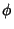as a sum of Orthonormal Functionssatisfying(1)

as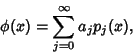(2)

and plug into(3)

giving(4)

But we wish this to hold for all degrees of approximation, so(5)(6)

Settingin (1) gives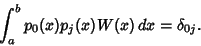(7)

The zeroth order orthonormal function can always be taken as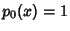, so (7) becomes(8)(9)

where (6) has been used in the last step. We therefore have the Matrix equation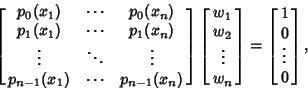(10)

which can be inverted to solve for thes (Press et al. 1992).

Abramowitz, M. and Stegun, C. A. (Eds.). Integration.'' §25.4 in Handbook of Mathematical Functions with Formulas, Graphs, and Mathematical Tables, 9th printing. New York: Dover, pp. 885-897, 1972.